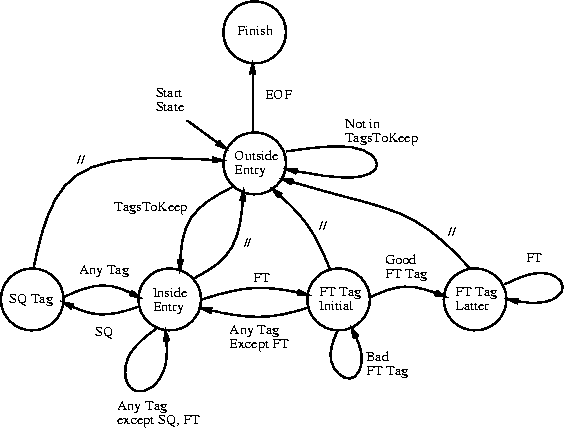Next: Map Files and Patricia Up: Genetic Databases Previous: Darwin Sequence Databases

# Building a Darwin Sequence Database

The previous section showed what a typical Darwin entry might look like. In this section, we build a rudimentary parsing engine to convert the entire contents of Swiss-Prot into a Darwin friendly format. The Darwin library offers the function SpToDarwin which performs a slightly extended version of what we perform here.

Calling Sequence:
SpToDarwin(flatfile, darwinfile, descr : name, compressed : boolean)
SpToDarwin(flatfile, darwinfile, descr : name)
Parameters:
 flatfile : name darwinfile : name descr : name compressed : boolean

Returns: Converts a Swiss-Prot flat file (flatfile) into a Darwin loadable file ( darwinfile). The parameter descr should contain a name item with the DESCR, DBNAME and DBRELEASE tags. If compressed is specified and true, flatfileis read using the Unix command zcat.

Our procedure, ConvertDB accepts either three or four parameters. The first argument flatfile is the flat file or raw data file containing all the Swiss-Prot entries we would like to convert. The second argument darwinfile is the destination file where we will store the converted form. The third argument allows us to specify the name of our database. The value of descript is wrapped in <DESCR>, </DESCR> tags. If the boolean variable compressed is specified and true, then flatfile is first decompressed via the UNIX zcat command.

After establishing a pipe between Darwin and the flat file flatfile (see §) and writing the database descriptor to file darwinfile, we can begin parsing the Swiss-Prot flat file. The parsing follows a simple schematic shown in Figure.ConvertDB := proc( flatfile : name, darwinfile : name,
descript : name, compressed : boolean )
local state;
secstruct := CreateString(1..5000);           # temporary holder for FT tag
state := 0;
# state  ->  0 : Outside Entry;  1 : Inside Entry;  2 : FT Tag Initial
#            3 : FT Tag Latter   4 : SQ Tag         5 : Finish
TagsToKeep := {'ID', 'AC', 'DE', 'OS', 'OC', 'FT', 'SQ'};

if compressed then                              # establish a pipe between Darwin and flatfile
OpenPipe('zcat '.flatfile);
else
fi;

WriteFile(darwinfile);
printf('<DBDESCR>%s<CONVDATE>%s</CONVDATE></DBDESCR>\n', descript, date());

t := ReadLine();              # get the first line from the file.
tag := t[1..2];               # 1st two characters contain the line type.
while (true) do               # we use break to get out of the loop.

if (state=0) then
if (t=EOF) then
state := 5;  next;
elif (member(tag, TagsToKeep)) then
state := 1; printf('\n<E>'); next;
# go to Inside Entry open an entry and skip to top
else
# do nothing                        # stay in state 0, ignore line
fi;
elif (state=1) then
if (tag='//') then
state := 0; printf('\n</E>');       # close entry
elif (tag='FT') then
state := 3; next;
elif (tag='SQ') then
state := 4; next;
elif (member(tag, TagsToKeep)) then
ParseTag(t); next;
fi;
elif (state=2) then
if (tag='FT') then
temp := ParseFTLine(t);
if (temp <> NULL) then            # information from the line was kept
state := 3; printf('\n<FT>%s', temp);  # good FT tag
fi;
elif (tag='//') then
state := 0; printf('\n</E>\n');
# go back Outside Entry and close entry
else
state := 1; next;                      # go back to Inside Entry
fi;
elif (state=3) then
if (agt='FT') then
for i to length(sec_struct) do sec_struct(i):=' '; od;
length_struct := 0;
ParseFTLine(t, sec_struct, length_struct);
elif (tag='//') then
state := 0;  printf('\n%s</FT></E>\n', sec_struct[1..length_struct]);
else
state :=1;   next;
fi;
elif (state=4) then
if (tag='SQ') then
printf('\n<SEQ>%s</SEQ>', ParseSequence();
next;
else
state := 1;  next;
fi;
elif (state=5) then
break;
fi;

t := ReadLine();             # get the next line from the pipe.
tag := t[1..2];              # get the next tag.
od;

WriteFile(terminal);
end:


All that remains from completing our parser is to write the procedures ParseTag, ParseSequence, and ParseFTLine.

 ParseTag := proc( t : name )
tag := t[1..2];
printf('\n<%s>', tag);
while (true) do
p:=3;
while (t[p] = ' ') do p := p+1 od;
printf('%s ', t[p]..length(t));
if (t[1..2] <> tag) then
printf('</%s>\n');
break;
fi;
od;
end;

ParseSequence := proc(  )
seq := '';                   # seq holds the partial sequence
while (true) do
t := ReadLine();           # get the next line from the pipe
tag := t[1..2];
if (tag = '  ') then
for p from 3 to length(t) do
if (t[p] > ' ') then
seq := seq . If(AToInt(t[p]) = 0, 'X', t[p]);
fi;
od;
else
return(seq);
fi;
od;
end;

ParseFTLine := proc( t : name, sec_struct : name, length_struct : posint )
global sec_struct, length_struct;

temp := sscanf(2+t, '%s %d %d');
if (length(temp) = 3) and ((temp='TURN') or (temp='HELIX')
or (temp='STRAND')) then
if (temp > length_struct) then length_struct := temp; fi;
for i from temp to temp do
if (temp='TURN') then sec_struct[i] := 't';
elif (temp = 'HELIX') then sec_struct[i] := 'h';
elif (temp = 'STRAND') then sec_struct[i] := 's';
fi;
od;
fi;
end;Next: Map Files and Patricia Up: Genetic Databases Previous: Darwin Sequence Databases
Gaston Gonnet
1998-09-15